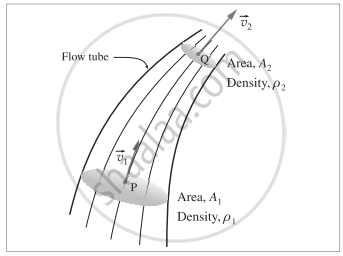Advertisement Remove all ads

# Obtain an expression for conservation of mass starting from the equation of continuity. - Physics

Answer in Brief

Obtain an expression for conservation of mass starting from the equation of continuity.

Advertisement Remove all ads

#### SolutionConsider a fluid in steady or streamline flow, that is its density is constant. The velocity of the fluid within a flow tube, while everywhere parallel to the tube, may change its magnitude. Suppose the velocity is vec"v"_1, at point P and vec"v"_2 at point Q. If A1 and A2 are the cross-sectional areas of the tube at these two points, the volume flux across A1, "d"/"dt"("V"_1) = "A"_1"v"_1
and that across A2,"d"/"dt"("V"_2) = "A"_2"v"_2

By the equation of continuity of flow for a fluid,

"A"_1"v"_1 = "A"_2"v"_2

i.e. "d"/"dt"("V"_1) = "d"/"dt"("V"_2)

If rho_1  "and"  rho_2 are the densities of the fluid at P and Q, respectively, the mass flux across "A"_1, "d"/"dt"("m"_1)

= "d"/"dt"(rho_1"V"_1) = "A"_1rho_1"v"_1

and that across A2, "d"/"dt"("m"_2) = "d"/"dt"(rho_2"V"_2) = "A"_2rho_2"v"_2

Since no fluid can enter or leave through the boundary of the tube, the conservation of mass requires the mass fluxes to be equal, i.e.,

"d"/"dt" ("m"_1) = "d"/"dt"("m"_2)

i.e. A1ρ1v1 = A2ρ2v2

i.e. Apv = constant which is the required expression.

Concept: Mechanical Properties of Fluids
Is there an error in this question or solution?
Advertisement Remove all ads

#### APPEARS IN

Balbharati Physics 12th Standard HSC Maharashtra State Board
Chapter 2 Mechanical Properties of Fluids
Exercises | Q 7 | Page 54
Advertisement Remove all ads
Advertisement Remove all ads
Share
Notifications

View all notifications

Forgot password?